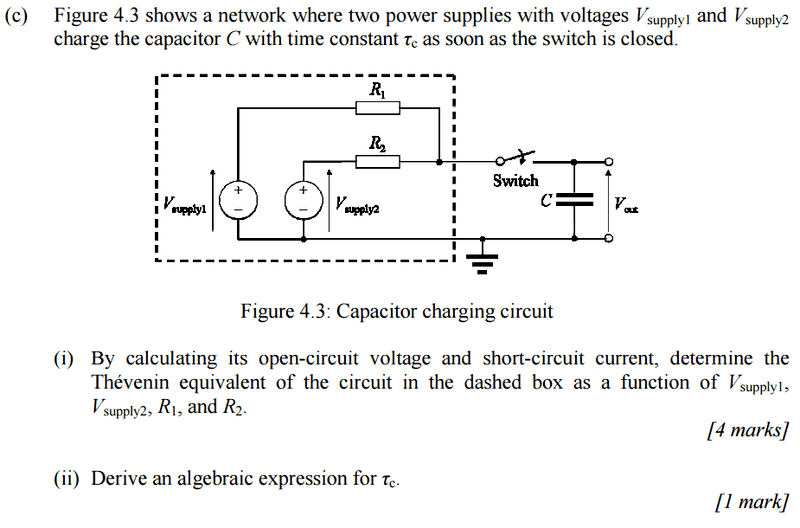# Determining Thevenin equivalent and time constant

• kev.thomson96
Having determined that, what will be the potential difference across R1?There's no current flowing, so it's R1/R2.3. Hence what will be the PD between the upper output of the dashed region and the positive terminal of Voltage Supply 1?PD=V1-V2=R1/(R1+R2)4. Then use that and the voltage of Voltage Supply 1 to calculate the PD between the negative terminal of Voltage Supply 1 and the upper output of the dashed region. That should be ##V_{Th}##, the Thevenin equivalent voltage of the dashed region.PD=V1-V2=R1/(

## Homework Statement## Homework Equations

; 3. The Attempt at a Solution [/B]

Here is my thought process and assumptions.

Without Vs1 and R2, the open-circuit current would be Vs2/R2, but I know Vs1 and R1 must be contributing in some way. I'm thinking of summing the Voltage sources and using the Potential divider to find Vopen-circuit. Can I do that?

I also assume we are calculating the open-circuit current and voltage after transforming into the Thevenin equivalent, not before (is this correct?), even though we are taking the circuit separately and an open-circuit is formed.

I know the time constant = RC, since after the Thevenin equivalent, we have RC circuit, driven by VThevenin.

kev.thomson96 said:
I'm thinking of summing the Voltage sources and using the Potential divider to find ##V_{open-circuit}##. Can I do that?
We can't just sum the voltages, as the cells are not in series. To calculate the open-circuit voltage, use the fact that the two -ve terminals are at the same potential and the PD between the two +ve terminals will be divided in the ratio R1 : R2 at the upper output terminal of the dashed region. So the open circuit voltage will be the voltage of one of the cells plus the appropriate share of the difference between the two cell voltages, as determined by that ratio.

andrewkirk said:
To calculate the open-circuit voltage, use the fact that the two -ve terminals are at the same potential and the PD between the two +ve terminals will be divided in the ratio R1 : R2 at the upper output terminal of the dashed region. So the open circuit voltage will be the voltage of one of the cells plus the appropriate share of the difference between the two cell voltages, as determined by that ratio.

So what you're saying is Vs1-Vs2=R1/R2 and Voc=Vs2R1/R2?

kev.thomson96 said:
So what you're saying is Vs1-Vs2=R1/R2
No
and Voc=Vs2R1/R2?
I don't know what you mean by ##V_{OC}##, but I doubt this is correct.

1. With the switch open, what will be the potential difference between the positive terminals of the two voltage supplies, as a function of ##V_{s1}## and ##V_{s2}##?

2. Having determined that, what will be the potential difference across R1?

3. Hence what will be the PD between the upper output of the dashed region and the positive terminal of Voltage Supply 1?

4. Then use that and the voltage of Voltage Supply 1 to calculate the PD between the negative terminal of Voltage Supply 1 and the upper output of the dashed region. That should be ##V_{Th}##, the Thevenin equivalent voltage of the dashed region.

•kev.thomson96
andrewkirk said:
I don't know what you mean by ##V_{OC}##, but I doubt this is correct.
Open-circuit
andrewkirk said:
1. With the switch open, what will be the potential difference between the positive terminals of the two voltage supplies, as a function of ##V_{s1}## and ##V_{s2}##?
Vs1/Vs2=R1/R2 since there's no current flowing?

I'm usually fine applying the potential divider to simple circuits, but the two power supplies are confusing me.

kev.thomson96 said:
Open-circuit

Vs1/Vs2=R1/R2 since there's no current flowing?

I'm usually fine applying the potential divider to simple circuits, but the two power supplies are confusing me.
The two voltage sources and the two resistors are in the same loop. You can apply KVL and get the voltage across R2, which is your Thevenin voltage(or Voc as you said). Assume a direction for current and proceed.

•kev.thomson96